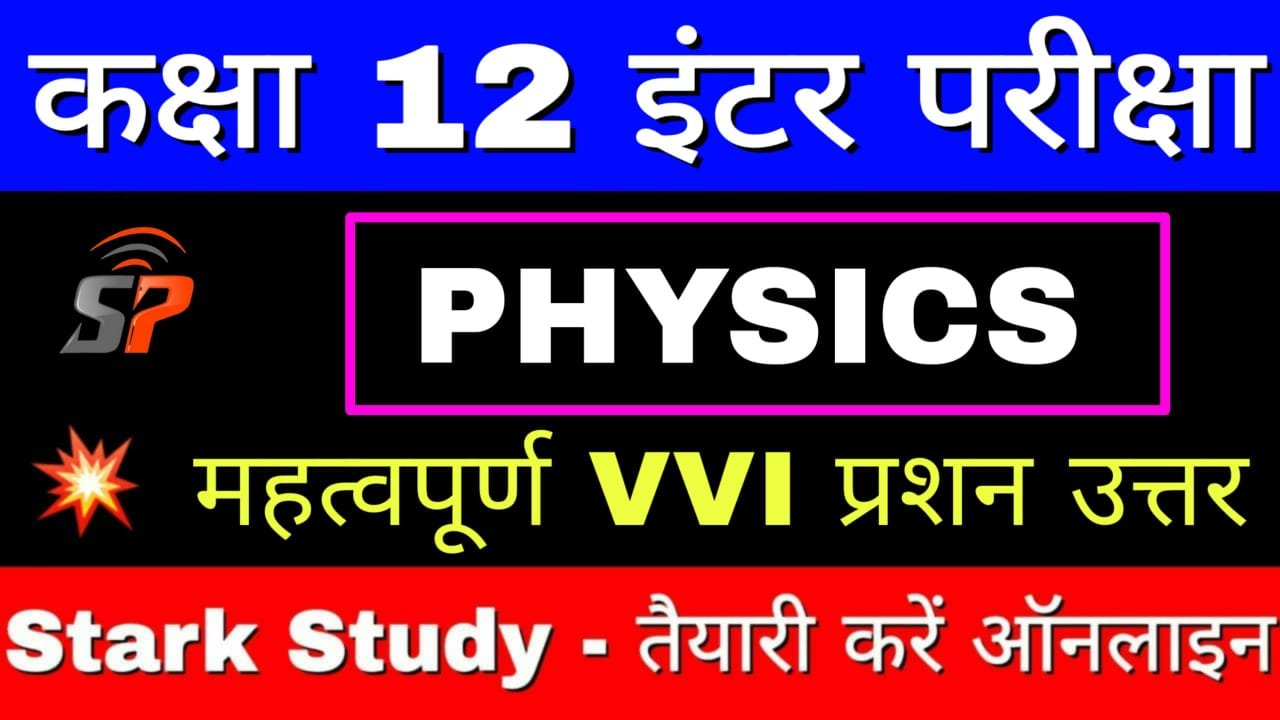Class 12th Physics Objective Question Nuclei Chapter on New Pattern

NUCLEI

1. Atomic number decreases with the emission of a particles from the nucleus :

(A) 1

(B) 2

(C) 3

(D) 4

2. Density of nucleus is about :

(A) 2.29 x 107 kg m-3

(B) 2.29 x 10-7 kg m-3

(C) 2.29 x 1017 kg m-3

(D) 2.29 x 10-17 kg m-3

3. Which of the relations is correct for radioactive atom ?

(A) Half-life = Average life

(B) Half-life = 2 x Average life

(C) Half-life = 1.6931 x Average life

(D) Half-life = 0.6931 x Average life

4. The equivalent energy of 1 g of substance is :

(A) 9 x 1013

(B) 6 x 1012 J

(C) 3 x 1013

(D) 6 x 1013 J

5. X-ray was discovered by:

(A) Becqueral

(B) Marie curie

(C) Roengton

(D) Vanlaw

6. The order of nuclear density is :

(A) 103

(B) 1017

(C) 106

(D) None

7. If R is the radius and A is the mass of a nucleus then the graph of log R versus log A will be:

(A) A straight line

(B) A parabola

(C) An Ellipse’

(D) None of these

8. The time in which radioactive substance become half of its intial amount is called :

(A) average life

(B) half-life

(C) time-period

(D) decay constant

9. Mass is converted into energy according to the relation :

(A) E = mc2

(B) E = mgh

(c) E = 1/2 mc

(D) E = mgh / c2

10. Who discovered heavy Hydrogen ?

(A) Uray

(B) Thomson

(C) Mosley

(D) Fermi

11. Which of the following is not a mode of radioactive decay ?

(A) positron emission

(B) electron capture

(C) fusion

(D) alpha decay

12. Which one of the following has maximum penetraing power:

(A) X-rays

(B) cathode rays

(C) α-rays

(D) Y-rays

13. Time during which the amount of radioactive substance becomes half of its initial amount is called.

(A) Average life

(B) Half life

(c) Decay constant

(D) Time period

14. β-rays are deflected in:

(A) Gravitational field

(B) Only in magnetic field

(C) Only in electrical field

(D) In magnetic and electric field both

15. Which one of following is charge less?

(A) Alpha particle

(B) Beta particle

(C) Photon particle

(D) Proton